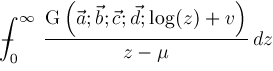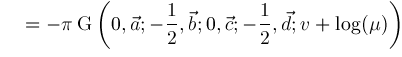﻿ Integration TheoremsISSAC 97 - Meijer G Function Representations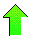Contents News View

 Integration Theorems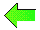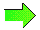Four theorems below are not original but serve as a small reference guide indicating the usefulness of the Meijer G function to solving integration problems.  These theorems are very general since many special functions can be represented as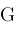functions.  We omit some rather complicated technical conditions on parameters which appear in the last three theorems pertaining to definite integration.  Readers may consult section 2.24 of Integrals and Series Volume 3: More Special Functions  for their complete statement and additional theorems.

Theorem.  (Indefinite Integration.)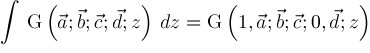Theorem.  (One G Function.)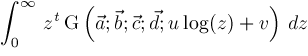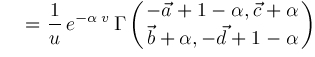where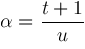Theorem.  (Two G Functions.)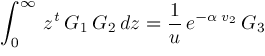where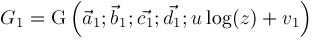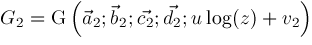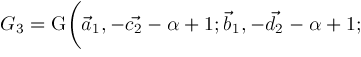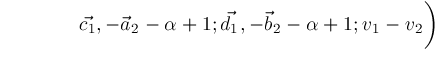Theorem.  (Cauchy Principal Value Integral.)